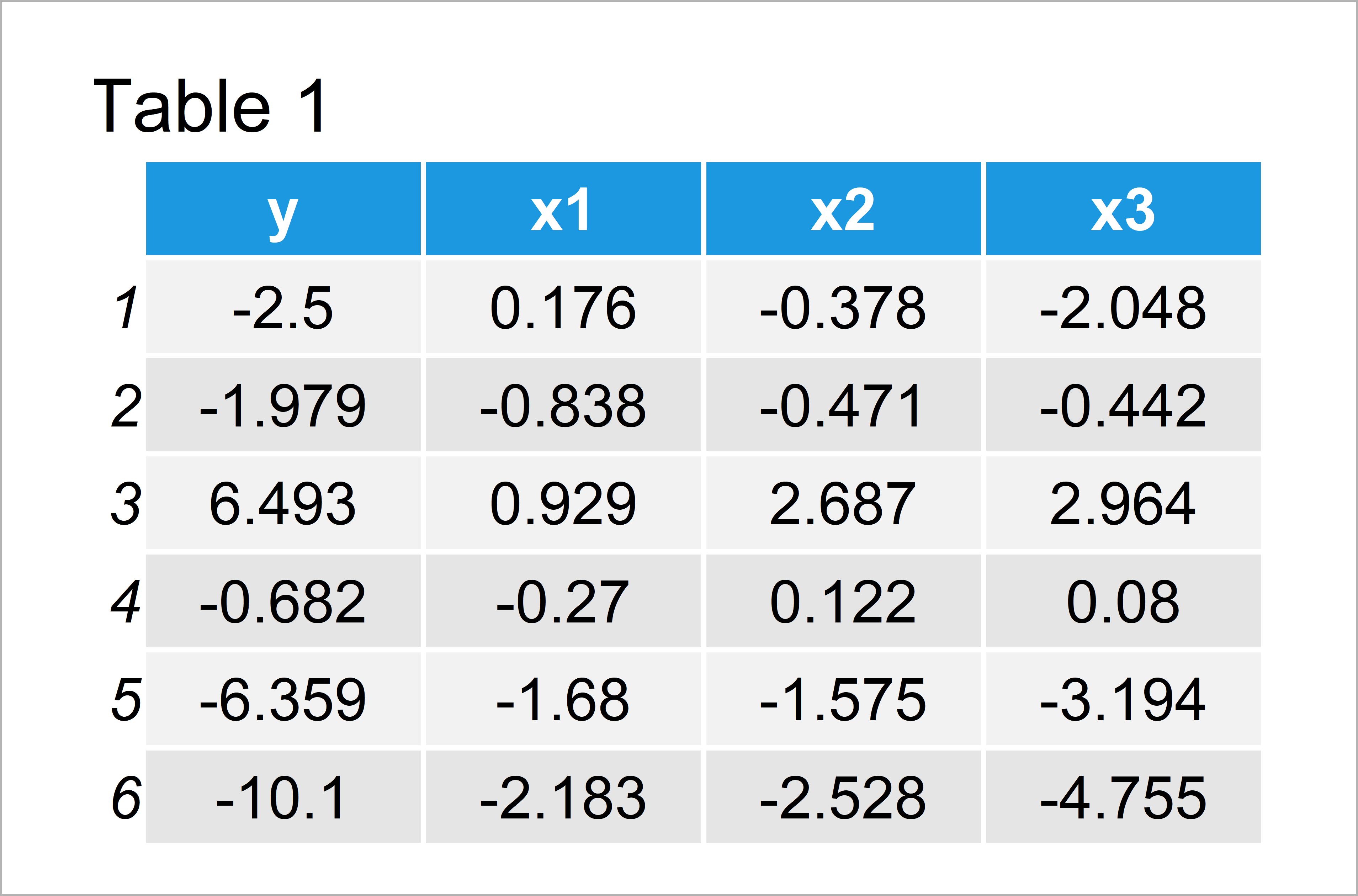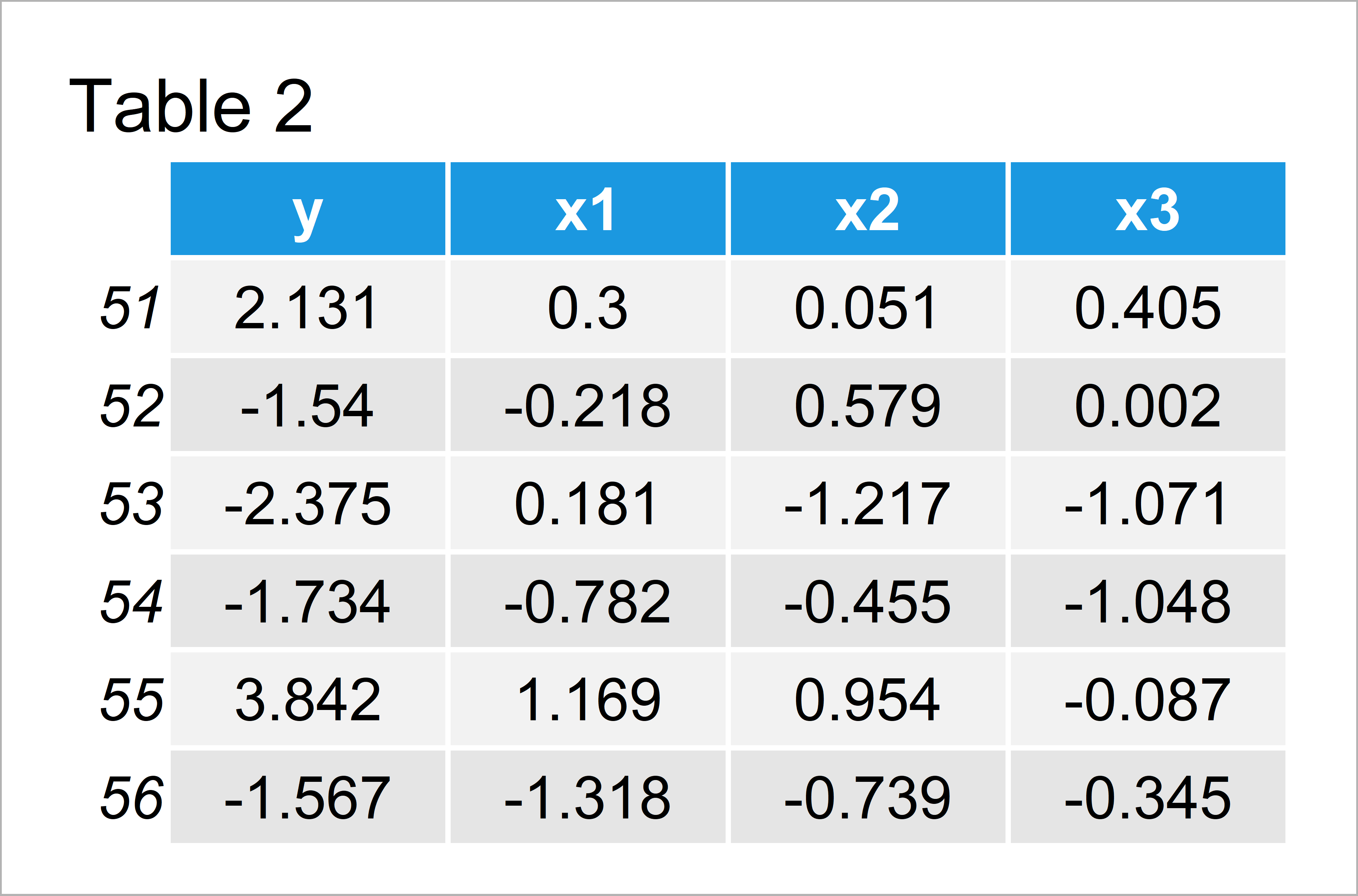# Error in model.frame.default : ‘data’ must be a data.frame, environment, or list

In this article, I’ll explain how to handle the error in model.frame.default: “‘data’ must be a data.frame, environment, or list” in R programming.

Let’s dig in…

## Example Data

The following random data is used as basement for this R programming tutorial:

```set.seed(5430987)                                # Create random example data
x1 <- rnorm(100)
x2 <- x1 + rnorm(100)
x3 <- x1 + 0.5 * x2 + rnorm(100)
y <- x1 + x2 + x3 + rnorm(100)```

Based on the previously created random vectors, we can create two data frames. The first data frame will be used for model estimation:

```data_1 <- data.frame(y, x1, x2, x3)[1:50, ]      # Create first data frameTable 1 shows the structure of our first example data – It contains 50 rows and four columns.

The second data frame will be used as input data for the predict function:

```data_2 <- data.frame(y, x1, x2, x3)[51:100, ]    # Create second data frameIn Table 2 you can see that we have managed to create a second data frame that also consists of 50 rows.

In the next step, we can estimate a linear model and create some summary statistics:

```my_mod <- lm(y ~ ., data_1)                      # Estimate linear model with first data
summary(my_mod)                                  # Summary statistics
#
# Call:
# lm(formula = y ~ ., data = data_1)
#
# Residuals:
#      Min       1Q   Median       3Q      Max
# -2.32650 -0.48689  0.06888  0.56707  2.36625
#
# Coefficients:
#             Estimate Std. Error t value Pr(>|t|)
# (Intercept)  -0.2174     0.1395  -1.558 0.126106
# x1            0.8836     0.2173   4.066 0.000185 ***
# x2            0.6291     0.1511   4.163 0.000136 ***
# x3            1.2494     0.1545   8.088  2.2e-10 ***
# ---
# Signif. codes:  0 ‘***’ 0.001 ‘**’ 0.01 ‘*’ 0.05 ‘.’ 0.1 ‘ ’ 1
#
# Residual standard error: 0.9493 on 46 degrees of freedom
# Multiple R-squared:  0.9226,	Adjusted R-squared:  0.9175
# F-statistic: 182.7 on 3 and 46 DF,  p-value: < 2.2e-16
#```

So far so good. Now, let’s assume that we want to use our model to predict the values in another data frame…

## Replicate the Error in model.frame.default : ‘data’ must be a data.frame, environment, or list

This example explains why the error message in “model.frame.default : ‘data’ must be a data.frame, environment, or list” can appear.

Have a look at the following R code:

```pred_values1 <- predict(my_mod, data_2\$y)        # Try to apply predict
# Error in model.frame.default(Terms, newdata, na.action = na.action, xlev = object\$xlevels) :
#   'data' must be a data.frame, environment, or list```

As you can see based on the previous output of the RStudio console, the error message in model.frame.default was returned.

The reason for this is that we didn’t specify the data argument properly, since we have used the column vector data_2\$y instead of the entire data frame.

Let’s solve this problem!

## Fix the Error in model.frame.default : ‘data’ must be a data.frame, environment, or list

The following R programming syntax illustrates how to avoid the error in model.frame.default: “‘data’ must be a data.frame, environment, or list”.

For this, we have to specify the data frame argument within the predict function properly, i.e. we have to insert a real data frame:

```pred_values2 <- predict(my_mod, data_2)          # Properly specify data argument
#        51        52        53        54        55        56
# -5.133051 -4.921293 -2.903784  3.134165 -2.959659 -2.093680```

The previous R syntax has returned predicted values for our second data frame. Looks good!

## Video, Further Resources & Summary

Do you need more information on the content of this tutorial? Then I recommend watching the following video of the Statistics Globe YouTube channel. I explain the topics of this tutorial in the video:

Please accept YouTube cookies to play this video. By accepting you will be accessing content from YouTube, a service provided by an external third party.If you accept this notice, your choice will be saved and the page will refresh.

Besides the video, you may want to read the related articles on this homepage.

In this R tutorial you have learned how to deal with the error in model.frame.default: “‘data’ must be a data.frame, environment, or list”. In case you have additional questions and/or comments, please let me know in the comments below.

Subscribe to the Statistics Globe Newsletter